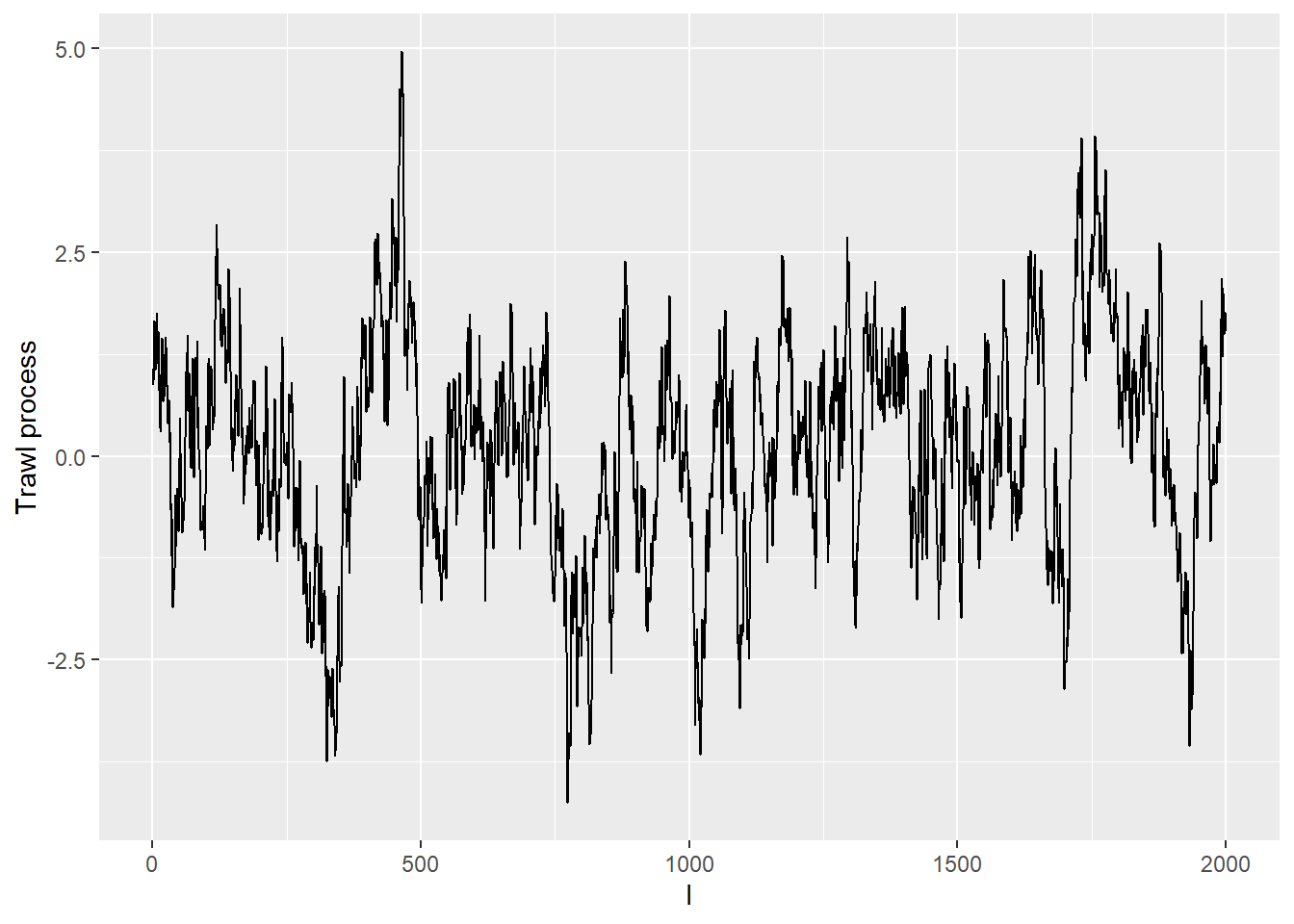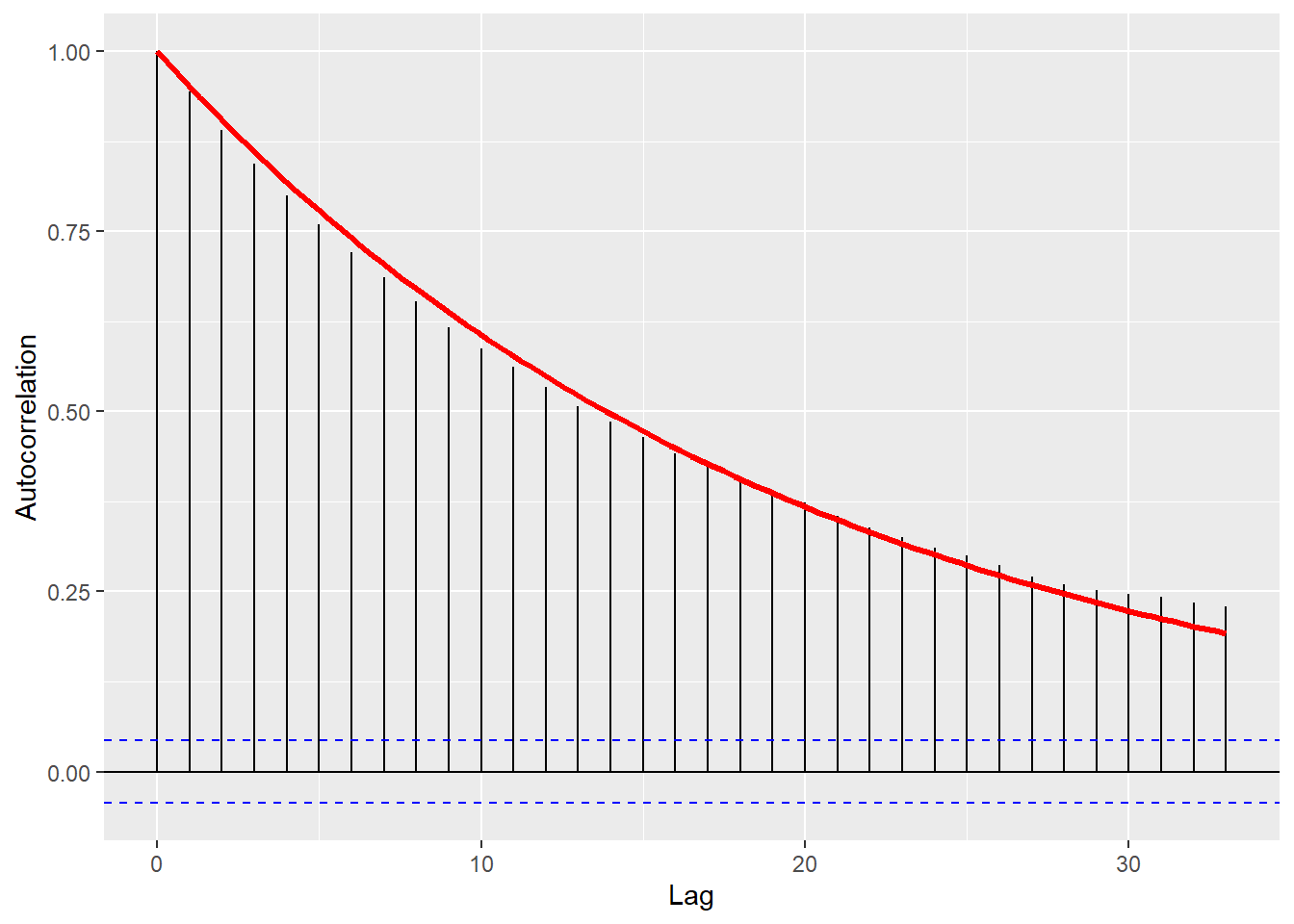# Definition of a (weighted) trawl process

The ambit package can be used to simulate univariate (weighted) trawl processes of the form $Y_t =\int_{(-\infty,t]\times \mathbb{R}} p(t-s)\mathbb{I}_{(0, a(t-s))}(x)L(dx,ds), \mbox{ for } t \ge 0.$ We refer to $$p$$ as the weight/kernel function, $$a$$ as the trawl function and $$L$$ as the Lévy basis.

If the function $$p$$ is given by the identity function, $$Y$$ is a trawl process, otherwise we refer to $$Y$$ as a weighted trawl process.

## Choice of the trawl function

This package only considers the case when the trawl function, denoted by $$a$$, is strictly monotonically decreasing.

The following implementations are currently included in the function sim_weighted_trawl:

• Exponential trawl function (“Exp”): The trawl function is parametrised by one parameter $$\lambda>0$$ and defined as

$a(x)=\exp(-\lambda x), \qquad \mathrm{for \,} x \geq 0.$

• supIG trawl function (“supIG”): The trawl function is parametrised by two parameters $$\delta$$ and $$\gamma$$, which are assumed to not be simultaneously equal to zero, and is defined as

$a(x)=(1+2x\gamma^{-2})^{-1/2}\exp(\delta \gamma(1-(1+2x\gamma^{-2})^{1/2})), \qquad \mathrm{for \,} x \geq 0.$

• supGamma trawl function (“LM”): The trawl function is parametrised by two parameters $$H> 1$$ and $$\alpha > 0$$, and is defined as

$a(x) = (1+x/\alpha)^{-H}, \qquad \mathrm{for \,} x \geq 0.$

Alternatively, the user can use the function sim_weighted_trawl_gen which requires specifying a monotonic trawl function $$a(\cdot)$$.

## Choice of kernel/weight

The user can choose a suitable weight function $$p$$. If no weight function is provided, then the function $$p(x)=1$$ for all $$x$$ is chosen. I.e. the resulting process is a trawl process rather than a weighted trawl process.

## Choice of Lévy bases

The driving noise of the process is given by a homogeneous Lévy basis denoted by $$L$$ with corresponding Lévy seed $$L'$$.

In the following, we denote by $$A$$ a Borel set with finite Lebesgue measure.

The following infinitely divisible distributions are currently included in the implementation:

### Support on $$(0, \infty)$$

• Gamma distribution (“Gamma”): $$L'\sim \Gamma(\alpha_g, \sigma_g)$$, where $$\alpha_g>0$$ is the shape parameter and $$\sigma_g>0$$ the scale parameter. Then the corresponding density is given by $f(x)=\frac{1}{\sigma_g^{\alpha_g}\Gamma(\alpha_{g})}x^{\alpha_g-1}e^{-x/\sigma_g},$ for $$x>0$$. The characteristic function is given by $\psi(u)=(1-ui\sigma_g)^{\alpha_g},$ for $$u\in \mathbb{R}$$. We note that $$\mathbb{E}(L')=\alpha_g \sigma_g$$, $$\mathrm{Var}(L')=\alpha_g \sigma_g^2$$ and $$c_4(L')=6\alpha \sigma^4$$. Here we have $L(A)\sim \Gamma(\mathrm{Leb}(A)\alpha_g, \sigma_g).$

### Support on $$\mathbb{R}$$

• Gaussian case (“Gaussian”): $$L'\sim \mathrm{N}(\mu, \sigma^2)$$. In this case, $L(A)\sim \mathrm{N}(\mathrm{Leb}(A)\mu, \mathrm{Leb}(A)\sigma^2).$ We note that $$\mathbb{E}(L')=\mu$$, $$\mathrm{Var}(L')=\sigma^2$$ and $$c_4(L')=0$$.

• Cauchy distribution (“Cauchy”): $$L'\sim \mathrm{Cauchy}(l, s)$$, where $$l\in \mathbb{R}$$ is the location parameter and $$s>0$$ the scale parameter. The corresponding density is given by $f(x)=\frac{1}{\pi s(1+(x-l)/s)^2}, \quad x \in \mathbb{R},$ and the characteristic function is given by $\psi(u)=l i u-s|u|, \quad u \in \mathbb{R}.$ Here we have $L(A) \sim \mathrm{Cauchy}(l\mathrm{Leb}(A), s\mathrm{Leb}(A)).$

• Normal inverse Gaussian case (“NIG”): $$L'\sim \mathrm{NIG}(\mu, \alpha, \beta, \delta)$$, where $$\mu \in \mathbb{R}$$ is the location parameter, $$\alpha \in \mathbb{R}$$ the tail heaviness parameter, $$\beta \in \mathbb{R}$$ the asymmetry parameter and $$\delta\in \mathbb{R}$$ the scale parameter. We set $$\gamma=\sqrt{\alpha^2-\beta^2}$$. The corresponding density is given by $f(x)=\frac{\alpha \delta K_1(\alpha\sqrt{\delta^2+(x-\mu)^2})}{\pi\sqrt{\delta^2+(x-\mu)^2}} \exp(\delta \gamma+\beta(x-\mu)), \quad x \in \mathbb{R}.$ Here $$K_1$$ denotes the Bessel function of the third kind with index 1. The characteristic function is given by $\psi(u)=\exp(iu\mu+\delta(\gamma-\sqrt{\alpha^2-(\beta+iu)^2})), \quad u \in \mathbb{R}.$ In this case, we have $L(A)\sim \mathrm{NIG}(\mu \mathrm{Leb}(A), \alpha, \beta, \delta \mathrm{Leb}(A)).$ Also, $$\mathbb{E}(L')=\mu +\frac{\delta \beta}{\gamma}$$, $$\mathrm{Var}(L')=\frac{\delta \alpha^2}{\gamma^3}$$ and $$c_4(L')=\frac{3\alpha^2\delta(4\beta^2+\alpha^2)}{\gamma^7}$$.

### Support on $$\mathbb{N}_0=\{0, 1, \ldots\}$$

• Poisson case (“Poisson”): $$L' \sim \mathrm{Poi}(v)$$ for $$v > 0$$. In this case, $L(A)\sim \mathrm{Poi}(\mathrm{Leb}(A)v).$ We note that $$\mathbb{E}(L')=\lambda$$, $$\mathrm{Var}(L')=\lambda$$ and $$c_4(L')=\lambda$$.

• Negative binomial case (“NegBin”): $$L'\sim \mathrm{NegBin}(m, \theta)$$ for $$m>0, \theta \in (0, 1)$$. I.e. the corresponding probability mass function is given by $$\mathrm{P}(L'=x)=\frac{1}{x!}\frac{\Gamma \left( m +x\right) }{\Gamma \left( m \right) }\left( 1-\theta \right)^{m }\theta^{x}$$ for $$x \in \{0, 1, \ldots\}$$. We note that $$\mathbb{E}(L')=m \theta/(1-\theta)$$, $$\mathrm{Var}(L')=m \theta/(1-\theta)^2$$ and $$c_4(L')=m\theta(\theta^2+4\theta+1)/(\theta-1)^4$$. Then,

$L(A)\sim \mathrm{NegBin}(\mathrm{Leb}(A)m, \theta).$

### Examples

We demonstrate the simulation of various trawl processes.

library(ambit)
library(ggplot2)

We start off with a trawl with standard normal marginal distribution and exponential trawl function.

#Set the number of observations
n <-2000
#Set the width of the grid
Delta<-0.1

#Determine the trawl function
trawlfct="Exp"
trawlfct_par <-0.5

#Choose the marginal distribution
distr<-"Gauss"
#mean 0, std 1
distr_par<-c(0,1)

#Simulate the path
set.seed(233)
path <- sim_weighted_trawl(n, Delta, trawlfct, trawlfct_par, distr, distr_par)$path #Plot the path df <- data.frame(time = seq(0,n,1), value=path) p <- ggplot(df, aes(x=time, y=path))+ geom_line()+ xlab("l")+ ylab("Trawl process") p#Plot the empirical acf and superimpose the theoretical one #Plot the acf my_acf <- acf(path, plot = FALSE) my_acfdf <- with(my_acf, data.frame(lag, acf)) #Confidence limits alpha <- 0.95 conf.lims <- c(-1,1)*qnorm((1 + alpha)/2)/sqrt(n) q <- ggplot(data = my_acfdf, mapping = aes(x = lag, y = acf)) + geom_hline(aes(yintercept = 0)) + geom_segment(mapping = aes(xend = lag, yend = 0))+ geom_hline(yintercept=conf.lims, lty=2, col='blue') + geom_function(fun = function(x) acf_Exp(x*Delta,trawlfct_par), colour="red", size=1.2)+ xlab("Lag")+ ylab("Autocorrelation") qThe same trawl process can be obtained using the sim_weighted_trawl_gen instead as follows: #Set the number of observations n <-2000 #Set the width of the grid Delta<-0.1 #Determine the trawl function trawlfct_par <-0.5 a <- function(x){exp(-trawlfct_par*x)} #Choose the marginal distribution distr<-"Gauss" #mean 0, std 1 distr_par<-c(0,1) #Simulate the path set.seed(233) path <- sim_weighted_trawl_gen(n, Delta, trawlfct_gen=a, distr, distr_par)$path

#Plot the path
df <- data.frame(time = seq(0,n,1), value=path)
p <- ggplot(df, aes(x=time, y=path))+
geom_line()+
xlab("l")+
ylab("Trawl process")
p#Plot the empirical acf and superimpose the theoretical one

#Plot the acf
my_acf <- acf(path, plot = FALSE)
my_acfdf <- with(my_acf, data.frame(lag, acf))
#Confidence limits
alpha <- 0.95
conf.lims <- c(-1,1)*qnorm((1 + alpha)/2)/sqrt(n)
q <- ggplot(data = my_acfdf, mapping = aes(x = lag, y = acf)) +
geom_hline(aes(yintercept = 0)) +
geom_segment(mapping = aes(xend = lag, yend = 0))+
geom_hline(yintercept=conf.lims, lty=2, col='blue') +
geom_function(fun = function(x) acf_Exp(x*Delta,trawlfct_par), colour="red", size=1.2)+
xlab("Lag")+
ylab("Autocorrelation")
q